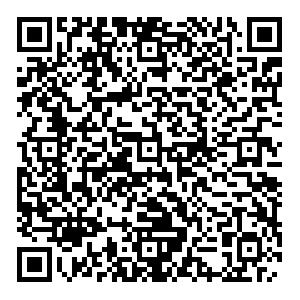# Effect of momentum anisotropy on quark matter in the quark-meson model• We investigate the chiral phase structure of quark matter with spheroidal momentum-space anisotropy specified by one anisotropy parameter $\xi$in the 2+1 flavor quark-meson model. We find that the chiral phase diagram and the location of the critical endpoint (CEP) are significantly affected by the value of $\xi$. With an increase in $\xi$, the CEP is shifted to lower temperatures and higher quark chemical potentials. In addition, the temperature of the CEP is more sensitive to the anisotropy parameter than the corresponding quark chemical potential, which is the opposite to that from the finite system volume effect. The effects of the momentum anisotropy on the thermodynamic properties and scalar (pseudoscalar) meson masses are also studied at the vanishing quark chemical potential. The numerical results reveal that an increase in $\xi$can hinder the restoration of chiral symmetry. We also find that shear viscosity and electrical conductivity decrease as $\xi$increases. However, the bulk viscosity exhibits a significant non-trivial behavior with $\xi$in the entire temperature domain of interest.
••Get Citation
He-Xia Zhang and Ben-Wei Zhang. Effect of momentum anisotropy on quark matter in the quark-meson model[J]. Chinese Physics C.
He-Xia Zhang and Ben-Wei Zhang. Effect of momentum anisotropy on quark matter in the quark-meson model[J]. Chinese Physics C.Milestone
Article Metric

Article Views(51)
Cited by(0)
Policy on re-use
To reuse of Open Access content published by CPC, for content published under the terms of the Creative Commons Attribution 3.0 license (“CC CY”), the users don’t need to request permission to copy, distribute and display the final published version of the article and to create derivative works, subject to appropriate attribution.
###### 通讯作者: 陈斌, bchen63@163.com
• 1.

沈阳化工大学材料科学与工程学院 沈阳 110142

Title:
Email:

## Effect of momentum anisotropy on quark matter in the quark-meson model

###### Corresponding author: Ben-Wei Zhang, bwzhang@mail.ccnu.edu.cn
• 1. Key Laboratory of Quark & Lepton Physics (MOE) and Institute of Particle Physics, Central China Normal University, Wuhan 430079, China
• 2. Institute of Quantum Matter, South China Normal University, Guangzhou 510006, China

Abstract: We investigate the chiral phase structure of quark matter with spheroidal momentum-space anisotropy specified by one anisotropy parameter $\xi$in the 2+1 flavor quark-meson model. We find that the chiral phase diagram and the location of the critical endpoint (CEP) are significantly affected by the value of $\xi$. With an increase in $\xi$, the CEP is shifted to lower temperatures and higher quark chemical potentials. In addition, the temperature of the CEP is more sensitive to the anisotropy parameter than the corresponding quark chemical potential, which is the opposite to that from the finite system volume effect. The effects of the momentum anisotropy on the thermodynamic properties and scalar (pseudoscalar) meson masses are also studied at the vanishing quark chemical potential. The numerical results reveal that an increase in $\xi$can hinder the restoration of chiral symmetry. We also find that shear viscosity and electrical conductivity decrease as $\xi$increases. However, the bulk viscosity exhibits a significant non-trivial behavior with $\xi$in the entire temperature domain of interest.

### HTML关注分享DownLoad:  Full-Size Img  PowerPoint